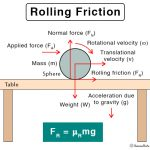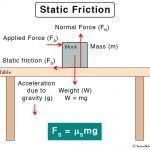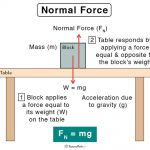Home / Physics / Magnetic Force

# Magnetic Force

## What is a Magnetic Force?

When a charged particle moves in a magnetic field with a specific velocity, it experiences a deflection due to a force acting on it. Aside, two current-carrying wires, when placed close to one another, share a similar force. It is known as a magnetic force and forms an integral part of electromagnetism. Magnetic force is non-contact. It can be both attractive and repulsive.

Two English scientists, Michael Faraday and James Clerk Maxwell, have made significant discoveries in electromagnetism.

## How is the Magnetic Force Measured?

### Magnetic Force Equation

Suppose a charge q is moving with a velocity v in a magnetic field of strength B, the formula for the magnetic force is

$\vec{F} = q\vec{v} \ X\ \vec{B}$

Here, F is represented as a vector, and v x B is the cross product of v and B. In terms of its magnitude, suppose θ is the angle between v and B, then

F = q v B sin θ

If v and B are at 90 degrees, then the above equation simplifies to

F = q v B

Unit of magnetic force: Newton or N.

Lorentz Magnetic Force

In addition to the magnetic field, if an electric field of strength E is present, then the force experienced by the particle is

$\vec{F} = q\vec{E}\ +\ q\vec{v} \ X\ \vec{B}$

The above equation is known as the Lorentz force equation.

### Right-Hand Rule

This rule is used to find the direction of magnetic force F on a positive moving charge using the forefinger, middle finger, and thumb at right angles to each other. According to this rule, if the right-hand thumb points in the direction of velocity v and the forefinger points in B's direction, the middle finger points to the force’s direction. In other words, F is perpendicular to the right-hand palm.

## Examples of Magnetic Force

Here are some examples of the magnetic force in different situations, along with the diagrams.

### 1. Current-carrying Wire

Moving charges in a wire give rise to current, defined as charge divided by time. Consider a conducting wire of length L carrying current I in a magnetic field of strength B then the magnetic force is given by

$\vec{F} = I\vec{L} \ X\ \vec{B}$

If the angle between the wire and the magnetic field is θ, then the magnitude of F is

F = I L B sin θ

This equation gives the relationship between the current and magnetic force.

### 2. Two Parallel Wires

Imagine two wires placed parallel to one another. The magnetic field at a distance r from an infinitely long wire carrying current I1 is given by

B1 = μo I1 / 2 π r

When a second wire carrying current I2 is brought closer and kept parallel to the first, it experiences a force given by

F = I2 L B1

Or, F = μo I1 I2 L / 2 π r

The force per unit length is

F/L = μo I1 I2  / 2 π r

The force is attractive if the currents are in the same direction and repulsive if they are opposite directions.

### 3. Two Magnets

Magnets exert forces on each other and other metals. We can see this example in daily life. The capability of a magnet to attract objects comes from the principles of electromagnetism. According to electromagnetic theory, electrons’ motion around nuclei results in a current called a magnetic dipole. An ordinary bar magnet consists of many permanent dipoles, whose interaction is the source of the magnetic force. It is possible to quantify this force by adding individual interactions.

A bar magnet consists of two poles located at the two ends. These ends are called the north pole and south pole. When two bar magnets are brought closer to one another, they experience a force of attraction or repulsion, depending on the nature of the poles. In general, like poles repel and opposite poles attract.

## Uses and Applications of the Magnetic Force

The magnetic force is applied in many devices and equipment. These include:

• Mass spectrometer
• Electromagnets
• AC and DC motors
• Transformers
• Maglev trains
• Magnetic Resonance Imaging

## Electric and Magnetic Forces

There are quite a few similarities and differences between electric and magnetic forces. Here are some of them.

• Caused by the attraction and repulsion of charges.
• Have lines of forces that originate from and terminate to a particular point
• Have dipoles
• Like poles/charges repel, opposite poles/charges attract

Here are some differences between the electrostatic and magnetic forces.

Article was last reviewed on Tuesday, November 17, 2020

### Related articlesRolling FrictionSliding FrictionStatic FrictionNormal Force